# 阴影映射

Note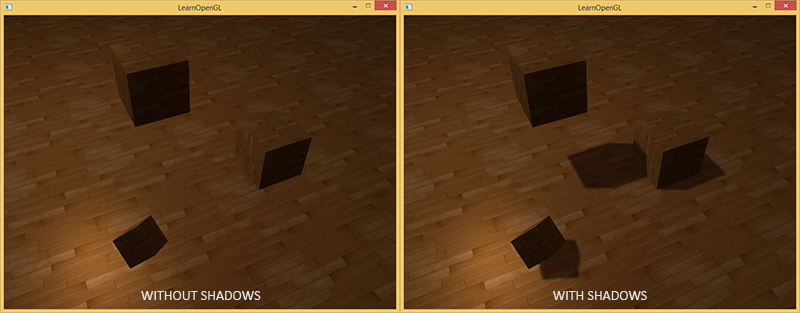## 阴影映射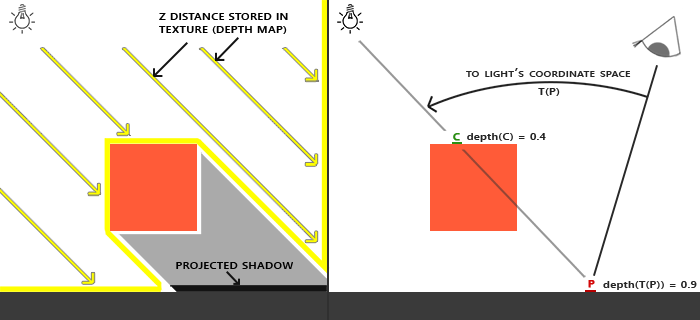Important

## 深度贴图

GLuint depthMapFBO;
glGenFramebuffers(1, &depthMapFBO);


const GLuint SHADOW_WIDTH = 1024, SHADOW_HEIGHT = 1024;

GLuint depthMap;
glGenTextures(1, &depthMap);
glBindTexture(GL_TEXTURE_2D, depthMap);
glTexImage2D(GL_TEXTURE_2D, 0, GL_DEPTH_COMPONENT,
glTexParameteri(GL_TEXTURE_2D, GL_TEXTURE_MIN_FILTER, GL_NEAREST);
glTexParameteri(GL_TEXTURE_2D, GL_TEXTURE_MAG_FILTER, GL_NEAREST);
glTexParameteri(GL_TEXTURE_2D, GL_TEXTURE_WRAP_S, GL_REPEAT);
glTexParameteri(GL_TEXTURE_2D, GL_TEXTURE_WRAP_T, GL_REPEAT);


glBindFramebuffer(GL_FRAMEBUFFER, depthMapFBO);
glFramebufferTexture2D(GL_FRAMEBUFFER, GL_DEPTH_ATTACHMENT, GL_TEXTURE_2D, depthMap, 0);
glDrawBuffer(GL_NONE);
glBindFramebuffer(GL_FRAMEBUFFER, 0);


// 1. 首选渲染深度贴图
glBindFramebuffer(GL_FRAMEBUFFER, depthMapFBO);
glClear(GL_DEPTH_BUFFER_BIT);
RenderScene();
glBindFramebuffer(GL_FRAMEBUFFER, 0);
// 2. 像往常一样渲染场景，但这次使用深度贴图
glViewport(0, 0, SCR_WIDTH, SCR_HEIGHT);
glClear(GL_COLOR_BUFFER_BIT | GL_DEPTH_BUFFER_BIT);
glBindTexture(GL_TEXTURE_2D, depthMap);
RenderScene();


### 光源空间的变换

GLfloat near_plane = 1.0f, far_plane = 7.5f;
glm::mat4 lightProjection = glm::ortho(-10.0f, 10.0f, -10.0f, 10.0f, near_plane, far_plane);


glm::mat4 lightView = glm::lookAt(glm::vec(-2.0f, 4.0f, -1.0f), glm::vec3(0.0f, 0.0f, 0.0f), glm::vec3(0.0f, 1.0f, 0.0f));


glm::mat4 lightSpaceMatrix = lightProjection * lightView;


### 渲染至深度贴图

#version 330 core
layout (location = 0) in vec3 position;

uniform mat4 lightSpaceMatrix;
uniform mat4 model;

void main()
{
gl_Position = lightSpaceMatrix * model * vec4(position, 1.0f);
}


#version 330 core

void main()
{
// gl_FragDepth = gl_FragCoord.z;
}


simpleDepthShader.Use();
glUniformMatrix4fv(lightSpaceMatrixLocation, 1, GL_FALSE, glm::value_ptr(lightSpaceMatrix));

glBindFramebuffer(GL_FRAMEBUFFER, depthMapFBO);
glClear(GL_DEPTH_BUFFER_BIT);
glBindFramebuffer(GL_FRAMEBUFFER, 0);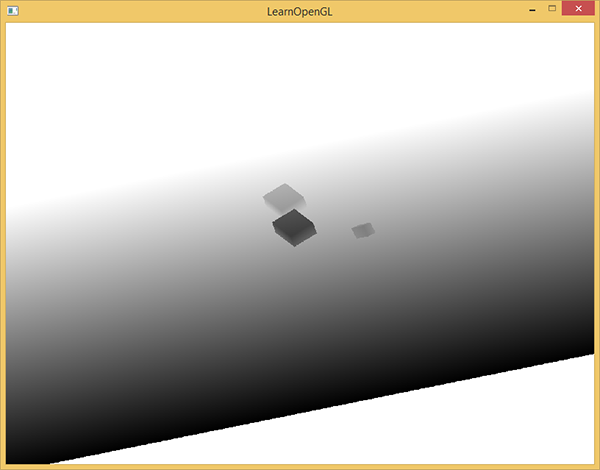#version 330 core
out vec4 color;
in vec2 TexCoords;

uniform sampler2D depthMap;

void main()
{
float depthValue = texture(depthMap, TexCoords).r;
color = vec4(vec3(depthValue), 1.0);
}


## 渲染阴影

#version 330 core
layout (location = 0) in vec3 position;
layout (location = 1) in vec3 normal;
layout (location = 2) in vec2 texCoords;

out vec2 TexCoords;

out VS_OUT {
vec3 FragPos;
vec3 Normal;
vec2 TexCoords;
vec4 FragPosLightSpace;
} vs_out;

uniform mat4 projection;
uniform mat4 view;
uniform mat4 model;
uniform mat4 lightSpaceMatrix;

void main()
{
gl_Position = projection * view * model * vec4(position, 1.0f);
vs_out.FragPos = vec3(model * vec4(position, 1.0));
vs_out.Normal = transpose(inverse(mat3(model))) * normal;
vs_out.TexCoords = texCoords;
vs_out.FragPosLightSpace = lightSpaceMatrix * vec4(vs_out.FragPos, 1.0);
}


#version 330 core
out vec4 FragColor;

in VS_OUT {
vec3 FragPos;
vec3 Normal;
vec2 TexCoords;
vec4 FragPosLightSpace;
} fs_in;

uniform sampler2D diffuseTexture;

uniform vec3 lightPos;
uniform vec3 viewPos;

{
[...]
}

void main()
{
vec3 color = texture(diffuseTexture, fs_in.TexCoords).rgb;
vec3 normal = normalize(fs_in.Normal);
vec3 lightColor = vec3(1.0);
// Ambient
vec3 ambient = 0.15 * color;
// Diffuse
vec3 lightDir = normalize(lightPos - fs_in.FragPos);
float diff = max(dot(lightDir, normal), 0.0);
vec3 diffuse = diff * lightColor;
// Specular
vec3 viewDir = normalize(viewPos - fs_in.FragPos);
vec3 reflectDir = reflect(-lightDir, normal);
float spec = 0.0;
vec3 halfwayDir = normalize(lightDir + viewDir);
spec = pow(max(dot(normal, halfwayDir), 0.0), 64.0);
vec3 specular = spec * lightColor;
// 计算阴影
vec3 lighting = (ambient + (1.0 - shadow) * (diffuse + specular)) * color;

FragColor = vec4(lighting, 1.0f);
}


float ShadowCalculation(vec4 fragPosLightSpace)
{
// 执行透视除法
vec3 projCoords = fragPosLightSpace.xyz / fragPosLightSpace.w;
[...]
}


Important

projCoords = projCoords * 0.5 + 0.5;


float closestDepth = texture(shadowMap, projCoords.xy).r;


float currentDepth = projCoords.z;


float shadow = currentDepth > closestDepth  ? 1.0 : 0.0;


float ShadowCalculation(vec4 fragPosLightSpace)
{
// 执行透视除法
vec3 projCoords = fragPosLightSpace.xyz / fragPosLightSpace.w;
// 变换到[0,1]的范围
projCoords = projCoords * 0.5 + 0.5;
// 取得最近点的深度(使用[0,1]范围下的fragPosLight当坐标)
// 取得当前片段在光源视角下的深度
float currentDepth = projCoords.z;
// 检查当前片段是否在阴影中
float shadow = currentDepth > closestDepth  ? 1.0 : 0.0;

}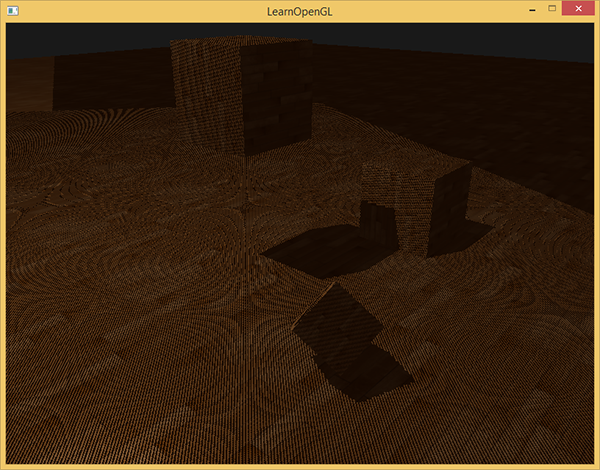## 改进阴影贴图

### 阴影失真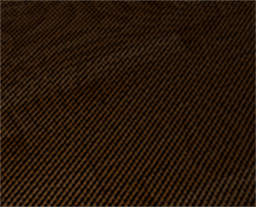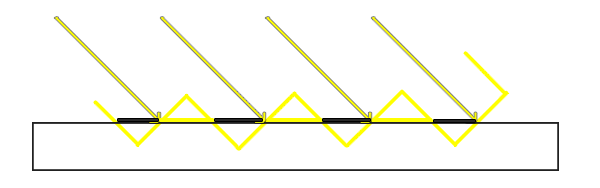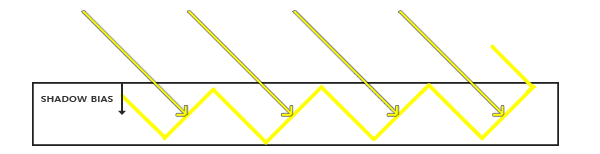float bias = 0.005;
float shadow = currentDepth - bias > closestDepth  ? 1.0 : 0.0;


float bias = max(0.05 * (1.0 - dot(normal, lightDir)), 0.005);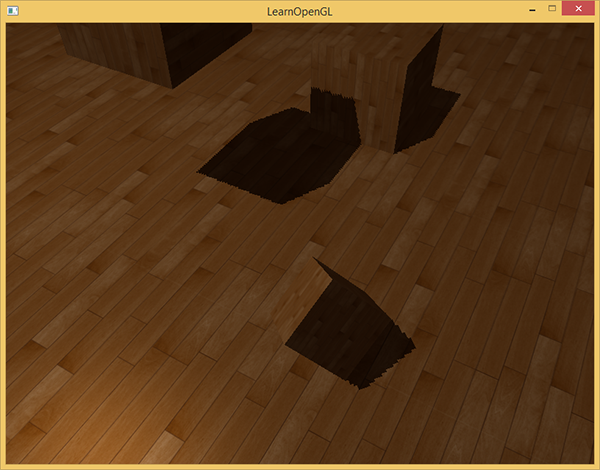### 悬浮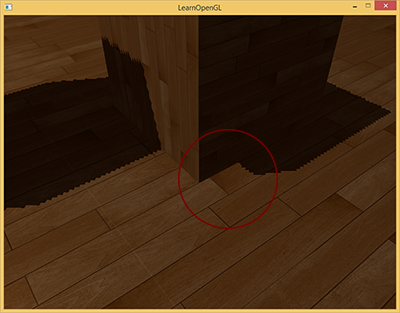glCullFace(GL_FRONT);
RenderSceneToDepthMap();
glCullFace(GL_BACK); // 不要忘记设回原先的culling face


### 采样过多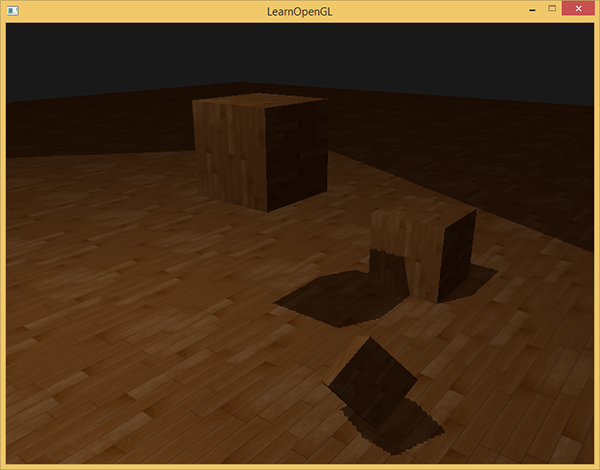glTexParameteri(GL_TEXTURE_2D, GL_TEXTURE_WRAP_S, GL_CLAMP_TO_BORDER);
glTexParameteri(GL_TEXTURE_2D, GL_TEXTURE_WRAP_T, GL_CLAMP_TO_BORDER);
GLfloat borderColor[] = { 1.0, 1.0, 1.0, 1.0 };
glTexParameterfv(GL_TEXTURE_2D, GL_TEXTURE_BORDER_COLOR, borderColor);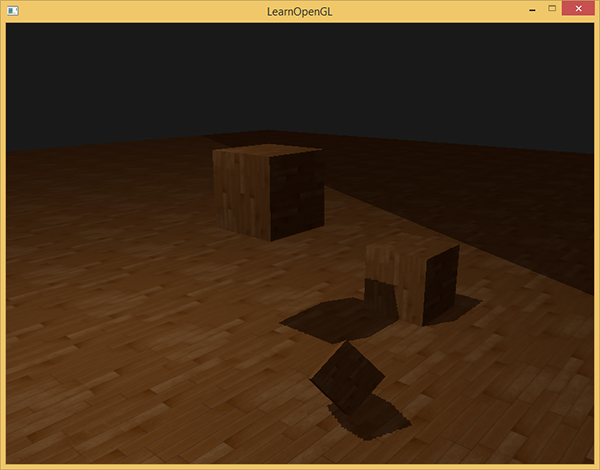float ShadowCalculation(vec4 fragPosLightSpace)
{
[...]
if(projCoords.z > 1.0)

}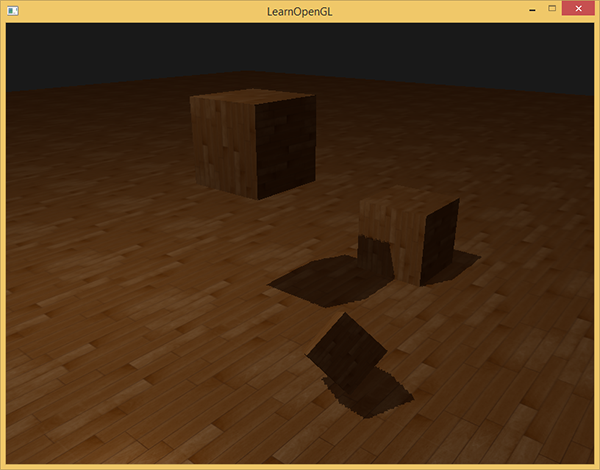## PCF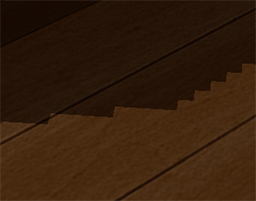float shadow = 0.0;
vec2 texelSize = 1.0 / textureSize(shadowMap, 0);
for(int x = -1; x <= 1; ++x)
{
for(int y = -1; y <= 1; ++y)
{
float pcfDepth = texture(shadowMap, projCoords.xy + vec2(x, y) * texelSize).r;
shadow += currentDepth - bias > pcfDepth ? 1.0 : 0.0;
}
}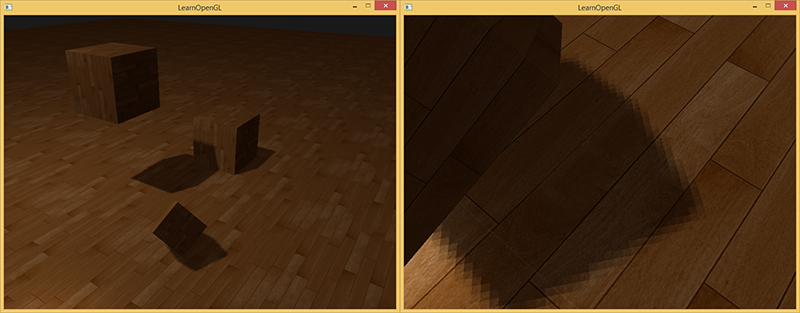### 正交 vs 投影#version 330 core
out vec4 color;
in vec2 TexCoords;

uniform sampler2D depthMap;
uniform float near_plane;
uniform float far_plane;

float LinearizeDepth(float depth)
{
float z = depth * 2.0 - 1.0; // Back to NDC
return (2.0 * near_plane * far_plane) / (far_plane + near_plane - z * (far_plane - near_plane));
}

void main()
{
float depthValue = texture(depthMap, TexCoords).r;
color = vec4(vec3(LinearizeDepth(depthValue) / far_plane), 1.0); // perspective
// color = vec4(vec3(depthValue), 1.0); // orthographic
}